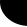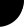HOME Electronics Directory Articles/ Tutorials eBooks About Us FORUM Links Contact UsVerilog Tutorial: Harsha Perla

Verilog Operators

Operators provide a means to construct expressions. Most of the operators are similar to that of C.

## List of operators:

 Arithmetic: +   --   *   / Modulus: % Relational: <   <=   >   >= Logical: !   &&   || Logical equality: ==   != Case equality: ===   !== Bit-wise: ~   &   |  ^    ~^   ^~ Reduction: &   ~&   |    ~|   ^   ~^   ^~ Shift: <<   >> Conditional: ? : Event or: or Concatenations: {}   {{}}

## Priority:

This list provides priority of Verilog Operators:

 Operator Type + - ! ~ Unary *  /  % Arithmetic +  -  (binary) Binary << >> Shift < <= > => Relational == != === !== Equality & ~& and nand ^ ~^ ^~ xor xnor | ~| or nor && Logical and || Logical or ?: Conditional operator

## Arithmetic operators

This table gives description of arithmetic operators.

 Operator Description a + b (Addition) a plus b a - b (Subtraction) a minus b a * b (Multiplication) a multiplied by b a / b (Division) a divide by b a % b (Modulus) a modulo b

The modulus operator is not allowed for real data type variables. Other arithmetic operators can be used with all data types. For the modulus operator, the result takes the sign of the first operand.

This code explains the usage of some basic operators:

 ```module operators(); reg [3:0] a, b; initial begin a = 3; b = 5; \$display(a + b ); // 8 \$display(a ? b: 1 ); // 5 \$display(a * b ); // 15 \$display(a / b ); // 0 \$display(b / a ); // 1 \$display(a % b ); // 3 \$display(7 % 3 ); // 1 \$display(7 % -3 ); // 1 \$display(-7 % 3 ); // -1 \$display(-7 % -3 ); // -1 end endmodule``` Output: 8 5 15 0 1 3 1 1 -1 -1

## Relational operators

The relational operators are used to compare expressions. The value returned by the relational operators is 0 if the expression evaluates to false and 1 if expression evaluates to true. Following table gives the list of relational operaors.

 Operator Description a < b a less than b a > b a greater than b a <= b a less than or equal to b a => b a greater than or equal to b

## Equality operators

The equality operators are used to compare expressions. If a comparison fails, then the result will be 0, otherwise it will be 1.

If both operands of logical equality (==) or logical inequality (!=) contain unknown (x) or a high-impedance (z) value, then the result of comparison will be unknown (x). Otherwise it will be 1 or 0.

If operands of case equality (===) or case inequality (!==) contain unknown (x) or a high-impedance (z) value, then the result will be calculated bit by bit.

## Logical operators

The logical operators are used to connect expressions.

 Operator Description a && b a and b a || b a or b !a not a

The result for these operators is 0 (when false), 1 (when true), and unknown (x - when ambiguous). The negation operator (!) turns a nonzero or true value of the operand into 0, zero or false value into 1, and ambiguous value of operator results in x (unknown value).

## Bit-wise operators

The bit-wise operators calculate each bit of results by evaluating the logical expression on a pair of corresponding operand bits.

 & 0 1 x z 0 0 0 0 0 1 0 1 x x x 0 x x x z 0 x x x

 | 0 1 x z 0 0 1 x x 1 1 1 1 1 x x 1 x x z x 1 x x

 ^ 0 1 x z 0 0 1 x x 1 1 0 x x x x x x x z x x x x

 ~^ ^~ 0 1 x z 0 1 0 x x 1 0 1 x x x x x x x z x x x x

 ~ Result 0 1 1 0 x x z x

## Reduction operators

The reduction operator produces a 1-bit result. This result is calculated by recursively applying bit-wise operation on all bits of the operand. At each step of this recursive calculation the logical bit-wise operation is performed on the result of a previous operation and on the next bit of the operand. The operation is repeated for all bits of the operand.

## Shift operators

The shift operators perform left and right shifts on their left operand by the number of positions specified by their right operand. All vacated bits are filled with zeroes. If the expression that specifies the number of bits to shift (right operand) has unknown (x) or high-impedance (z) value, then result will be unknown.

Examples of using the shift operators are in shown Example 7.

## Conditional operator

The conditional operator is described in the Conditional operator section.

## Concatenations

Concatenations are described in the Concatenations section.

## Event or operator

The event or operator is described in the section on Procedural timing controls.

## Example:

 ```module example(); reg [3:0] a, b; reg [7:0] c, d; initial begin a = 4'b1110; //14 b = 4'b0110; //5 \$display( "%b", a < b );// false - 0 \$display( "%b", a > 8 );// true - 1 \$display( "%b", a <= b );// false - 0 \$display( "%b", a >= 10 );// true - 1 \$display( "%b", a < 4'b1zzz );// unknown - x \$display( "%b", b < 4'b1x01 );// unknown - x a = 4'b1100; b = 4'b101x; \$display( "%b", a == 4'b1100 ); // true - 1 \$display( "%b", a != 4'b1100 );// false - 0 \$display( "%b", a == 4'b1z10 );// false - 0 \$display( "%b", a != 4'b100x );// true ? 1 \$display( "%b", b == 4'b101x );// unknown - x \$display( "%b", b != 4'b101x );// unknown - x \$display( "%b", b === 4'b101x );// true - 1 \$display( "%b", b !== 4'b101x );// false - 0 a = 4'b1100; b = 4'b0000; \$display( "%b", !a );// 0 - false \$display( "%b", !b );// 1 - true \$display( "%b", a && b ); // 0 - false \$display( "%b", a || b );// 1 ? true c = 8'b1010xzxz; d = 8'b10010011; \$display( "%b", c & d ); //= 8'b100000xx; \$display( "%b", c | d ); //= 8'b1011xx11; \$display( "%b", c ^ d ); //= 8'b0011xxxx; \$display( "%b", c ~^ d ); //= 8'b1100xxxx; \$display( "%b", ~ c ); //= 8'b0101xxxx; a = 4'b1111; \$display( "%b", a << 3 ); //= 4'b1000 \$display( "%b", a >> 3 ); //= 4'b0001 \$display( "%b", a << 1'bz ); //= 4'bxxxx \$display( "%b", a >> 1'bx ); //= 4'bxxxx end endmodule```

 value & ~& | ~| ^ ~^ ^~ 4'b0000 0 1 0 1 0 1 1 4'b0001 0 1 1 0 1 0 0 4'b0011 0 1 1 0 0 1 1 4'b0111 0 1 1 0 1 0 0 4'b1111 1 0 1 0 0 1 1 4'b01xx 0 1 1 0 x x x 4'b01z0 0 1 1 0 x x x

Reduction operators

Prev. : Verilog Datatypes
Verilog: Table of Contents
Ch:  1. 2. 3. 4. 5. 6. 7. 8. 9. 10. 11. 12. 13.
Next: Asynchronous
Counter example: initial and always block

 Home   |    About Us   |   Articles/ Tutorials   |   Downloads   |   Feedback   |   Links   |   eBooks   |   Privacy Policy Copyright © 2005-2007 electroSofts.com. webmaster@electroSofts.com